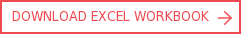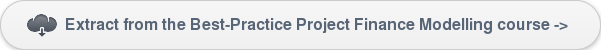View More Content
SHARE

PLCR – Project Life Cover RatioCategoriesTutorialsThe project life coverage ratio (PLCR) is a commonly used debt metric in project finance. Together with the debt service coverage ratio (DSCR) and loan life coverage ratio (LLCR), these debt metrics, in one form or another, usually appear in project finance term sheets and loan documentation, so they need to be modelled clearly and accurately.

The PLCR is akin to LLCR – it is the ratio of the net present value (NPV) of the cash flow over the remaining full life of the project to the outstanding debt balance in the period.This tutorial, and the accompanied workbook, cover the definition of the PLCR, show how to calculate this ratio, and highlight key points to keep in mind when modelling PLCR.

Definition of PLCR in a project finance model

Generally the PLCR is calculated as:

• PLCR = X / Y, where:

• X = NPV [ CFADS over project life ]

• Y = debt balance b/f

Unlike the LLCR, where the cash flow available for debt service (CFADS) is calculated over the scheduled life of the loan, the cash flow for PLCR is calculated over the ‘project life’.

In practice, lenders often build in a safety cushion and ignore the revenue or cash flow beyond a certain cut-off date. This allows lenders to protect against relying on potentially more uncertain future cash flows. It is common to encounter an end date for the PLCR calculation in the term sheet or loan documentation before the end of the project life. This means the qualifying period for CFADS in the PLCR calculation is only from commercial operation date (COD), and up to such end date.

The example in the workbook assumes the end date for the PLCR calculation is 12 months before the end of the operations.

Financial modelling of PLCR

Calculation of PLCR is similar to that of LLCR, with certain differences as highlighted below.Screenshot 1: Calculation of PLCR

Step 1 - Calculate the discount rate (‘r’)

This is the rate for discounting the CFADS. Similar to the LLCR, a typical discount rate would be the cost of debt, and can be calculated efficiently as:

r = interest / debt balance B/f

Note that the CFADS qualified for PLCR calculation extends beyond the loan life. So, what is the discount rate to be used post loan final maturity date? This depends upon a lenders’ perception of the certainty risk of the projected cash flows beyond the loan life.

Loan documentation often states the discount rate to be used post the loan life, which should be at least equal or even greater to the cost of debt of the senior debt at final maturity date, to account for greater risk. Let’s face it, if it was of similar perceived why not lend out that far?

Our simple example assumes a discount rate for the PLCR calculation post final maturity debt (final maturity of senior debt is 30-Jun-15) to be 8.00% p.a. or 1.94% p.q.

Step 2 - Create binary flag for Project Life

PLCR takes into account the NPV of CFADS over the life of a project or, if otherwise, a specified cut-off date (end date).

The end date for the PLCR calculation is usually stated in the term sheet or loan documentation, or else it should be the end of the operations date.

The qualifying CFADS for PLCR calculation shall then include the period from start of operations or commercial operation date (COD) up to the end date.

Step 3 - Calculate CFADS (NPV)

This is similar to the LLCR calculation, except that the CFADS is taken into account up to the end date as explained in Step 2 above.

Step 4  - Calculate PLCR

Bring in the CFADS (NPV) that has been calculated in Step 3 and the debt balance b/f.

PLCR shall then be calculated using formula below:

PLCR = CFADS (NPV) / debt balance b/f

Variations in the PLCR definition

Similar to the LLCR definition, it is not uncommon to find the balance of the project’s cash account, or the debt service reserve account (DSRA), added to the numerator or netted from the numerator. Note that extreme caution needs to be applied when assessing the economics of a project where the PLCR is supported with cash account balances.

When the DSRA is included, the PLCR is calculated as:

PLCR = (NPV [CFADS over project life] + DSRA/c balance b/f) / debt balance b/f

Common mistakes in the PLCR calculation

Discount rate post loan final maturity date

It is a common mistake to model the discount rate post loan final maturity date to be 0.00%, because the modelled cost of debt post the final maturity date is nil or just the base rate.

Note that the projected cash flows beyond the loan life are even more uncertain to be relied upon. Therefore, they should be discounted with the rate at least equal to cost of debt of the senior debt at the final maturity date.

One school of thought is to add the default margin to the cost of debt. The alternative is to leave it at the cost of debt on the basis it could be refinanced. The reality is that it makes little difference, as it is so far out for long term financings.

Should the PLCR always be higher than LLCR?

Common sense would indicate that the PLCR should be higher than the LLCR, owing to a longer evaluation period.

Be careful, the PLCR could be less than the LLCR, because projects particularly in mining or resource industries often have a substantial mine rehab cost or closure costs towards the end of the project life which can dramatically reduce the CFADS (the numerator for PLCR).Corality Training Academy - SMART CAMPUS

There are numerous other tutorials and free resources related to financial modelling in Corality's SMART Campus.

Some of the more popular courses that relate to this topic include:by Rickard Wärnelid

Rickard's passion for financial modelling is built on specialist roles in the highly quantitative fields of derivatives and project finance, a career path complemented by an academic grounding in engineering physics. Born in Sweden and with global consulting and leadership experience, Rickard is an internationally recognised authority, speaker and thought-leader on the organisational benefits of best practice financial modelling.

view all